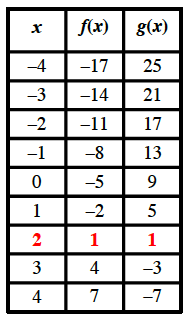Home > INT3 > Chapter 1 > Lesson 1.2.1 > Problem1-66

1-66.

Use any method to determine the point(s) of intersection of the graphs of $f(x) = 3x - 5$ and $y = g(x)$, shown in the table below. 1-66 HW eTool (Desmos). Homework Help ✎

 $x$ $-3$ $-2$ $-1$ $0$ $1$ $2$ $3$ $g(x)$ $21$ $17$ $13$ $9$ $5$ $1$ $-3$

Method 1: Graphing

Use the eTool below to find the point of intersection.
Click the link at right for the full version of the eTool: INT3 1-66 HW eTool

Method 2: Make a table.

Add values for the function $f(x)$ and compare values. Then check your answer in both equations.

point of intersection $= (2, 1)$